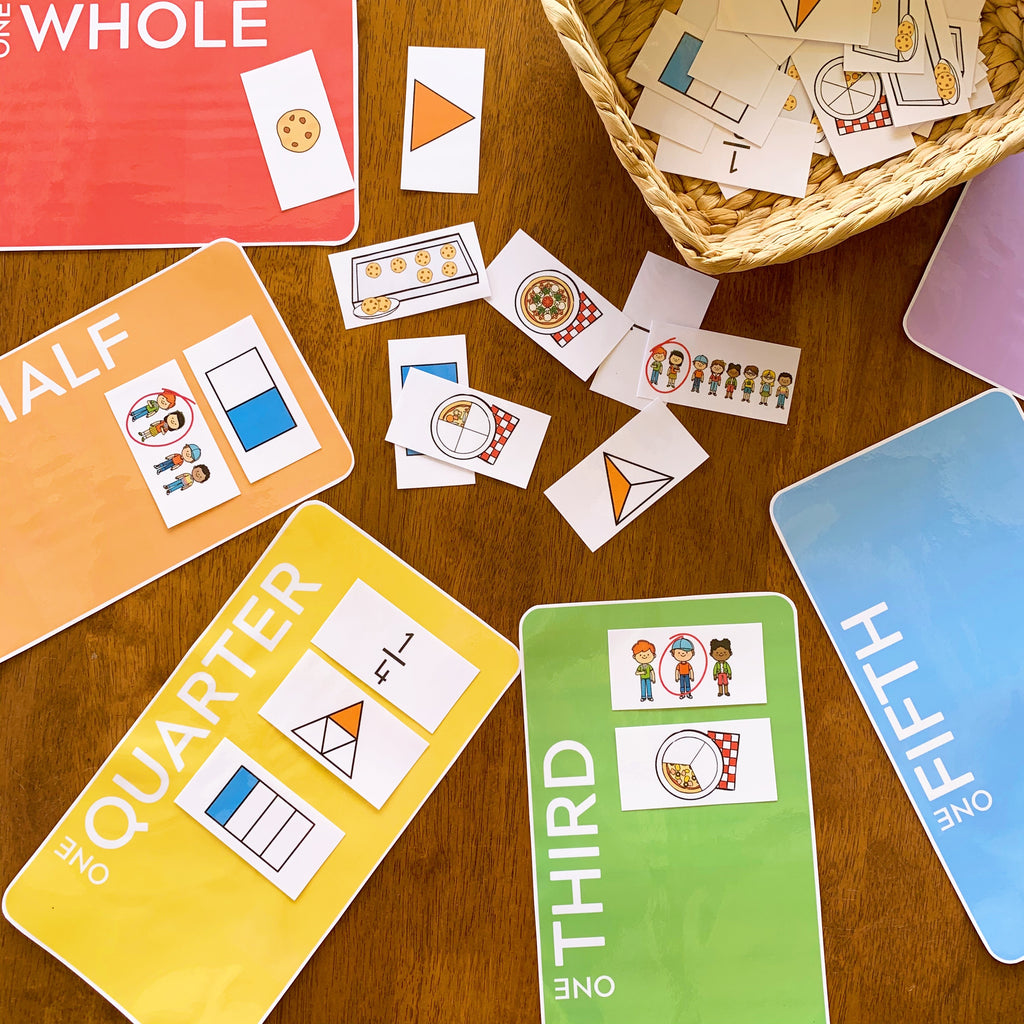# Fractions Sorting Pack

\$4.00

Learning about fractions? This sorting resource is ideal for children learning about the different ways fractions can be represented.

With multiple representations included for fractions – whole, half, quarters, thirds, eighths and more, pick and choose the cards you want your children to sort. Equivalent fractions, decimals and percentages have also been included for some of the fractions.

Alternatively, use these to create posters to display in class to help support your children and their understanding of fractions.

Here's what's included -

• fraction mats - one whole, one half, one quarter, one third, one fifth, one sixth, one eighth and one tenth - 1 per A4 page
• 6 different representations of each fraction (written fraction - numerator/denominator, rectangular shape divided into the fraction, pizza fractions, people as a fraction, alternative shape divided into the fraction, cookies as a fraction - 1 set of cards per A4 page
• 6 different representations of each fraction (written fraction - numerator/denominator, rectangular shape divided into the fraction, pizza fractions, people as a fraction, alternative shape divided into the fraction, cookies as a fraction - 2 set of cards per A4 page

To use -

You will need to have an up to date version of a PDF Reader like Adobe Reader installed on your computer to open this resource.